Chetaev theorems

(diff) ← Older revision | Latest revision (diff) | Newer revision → (diff)

Chetaev's theorems on stability are general theorems about the instability of motion, established by N.G. Chetaev for the equations of a perturbed motion of the form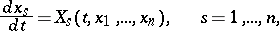(1)

in which the right-hand sidesare holomorphic functions of the real variableswith coefficients that are continuous bounded functions of a real variable — the time— defined on some domain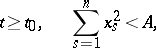(2)

where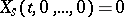.

Chetaev's theorem on instability of motion. The functionswhich turn up in the theory below are supposed to be real-valued functions of the variablesand, single valued and continuous in the domain

and with single-valued and continuous total time derivatives in the domainoccurring below forsmall enough,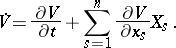It is also supposed that. Suppose that for the reduction of the system

to a domainthere exists a function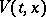such that its domain of positivityhas a non-empty open section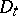contiguous with the coordinate origin 0 for all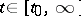, while on the part of the boundary of the domainlying inside the cylinder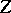and including the axisthere holds the equality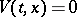. Now if 1) the function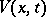is bounded on the domain; 2) the total derivativeis positive on this domain; and 3) for each subdomain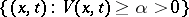there holds an inequality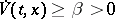, where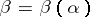is some positive number depending on, then the trivial solution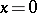of

is instable in the sense of Lyapunov for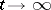.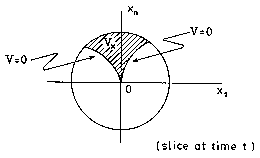Figure: c022080a

There are various other versions of this theorem. For example, a version involving two functions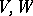, cf. . Still another version can be found in , p. 103. These theorems imply the so-called first instability theorem of Lyapunov, which says that if there exists a function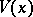with a negative-definite total derivative and withitself either negative definite or indefinite, then the equilibrium is unstable.

Chetaev's theorem on perturbations of stable motions of Hamiltonian systems. This Chetaev theorem is a theorem on the properties of the Poincaré variational equations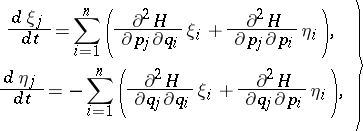(3)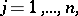for an unperturbed motion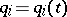,, under the assumption that the coefficients of the equations (3) are all continuous bounded real functions of,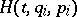is the Hamiltonian, and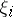andare the deviations of the coordinatesand the momenta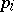. The equations (3) are of great significance in studies on the stability of the motion of conservative holonomic systems.

Theorem) If the unperturbed motion of a holonomic potential system is stable, then the characteristic numbers of all solutions of the variational equations (3) are equal to zero, the equations (3) are regular in the sense of Lyapunov, reduce to a system of equations with constant coefficients and have a quadratic integral of definite sign.

Chetaev's theorem generalizes Lagrange's theorem on an equilibrium and the Poincaré–Lyapunov theorem on a periodic motion. According to the theorem, for a stable unperturbed motion of a potential system, an infinitely near perturbed motion has an oscillatory, wave-like, character. From this Chetaev drew the conclusion that if there is an analogy between dynamics and Cauchy's mathematical theory of light, then one should look for it in perturbed motions close to stable motions of potential systems. And Chetaev found such an analogy (cf. [1e]), showing that a necessary condition for the stability of a holonomic conservative system leads to the wave equation. The optic-mechanical analogy was completely investigated by Chetaev, in the light also of the theory of Lie groups, based on replacing the original idea of the existence of an analogy between the two phenomena by that of the identification of the group of transformations of one phenomenon (the oscillatory process of diffusion of light) with that of other phenomenon (perturbed motion of a conservative system close to its stable motion). Chetaev proved (cf. [1f]) that this latter group is a unimodular group of linear transformations and has a presentation in the full group of Lorentz transformations, which is fundamental to the theory of light of Cauchy and Maxwell.